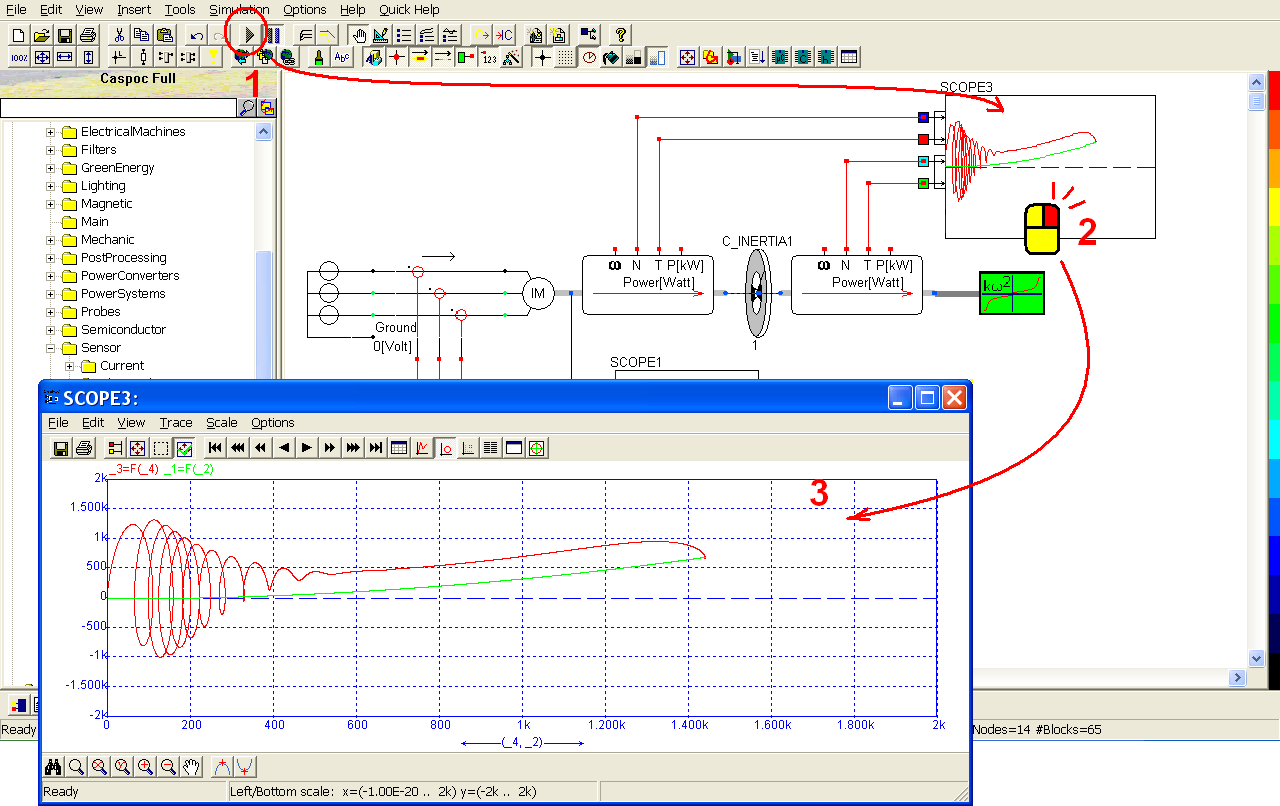## Induction Machine

Besides DC motor, AC motor is also becoming popular. There are many kinds of AC motors for different applications. The two main categories are induction motors and synchronous motors. In this example, we will use an induction motor to drive a variable load according a given 3-phase AC voltage source. The configuration is shown as below: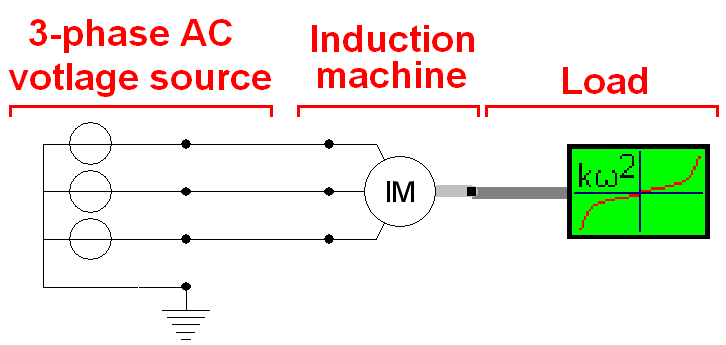To get the AC voltage source, left-click Components/Library/Source/Circuit/ThreePhase/VoltageSourceRMS (step 1) and release the mouse. The 3-phase AC voltage source follows the cursor and right-click to change its direction if needed (step 2). While all of its electrical round dots are rightward, left-click to put it on the workscreen. Right-click on the AC voltage source to check its setting (step 3). Then we can see the parameters of the AC voltage source: Frequency = 50 Hz, Phase = 0 and Vrms = 230 (step 4). Click OK to save the setting (step 5).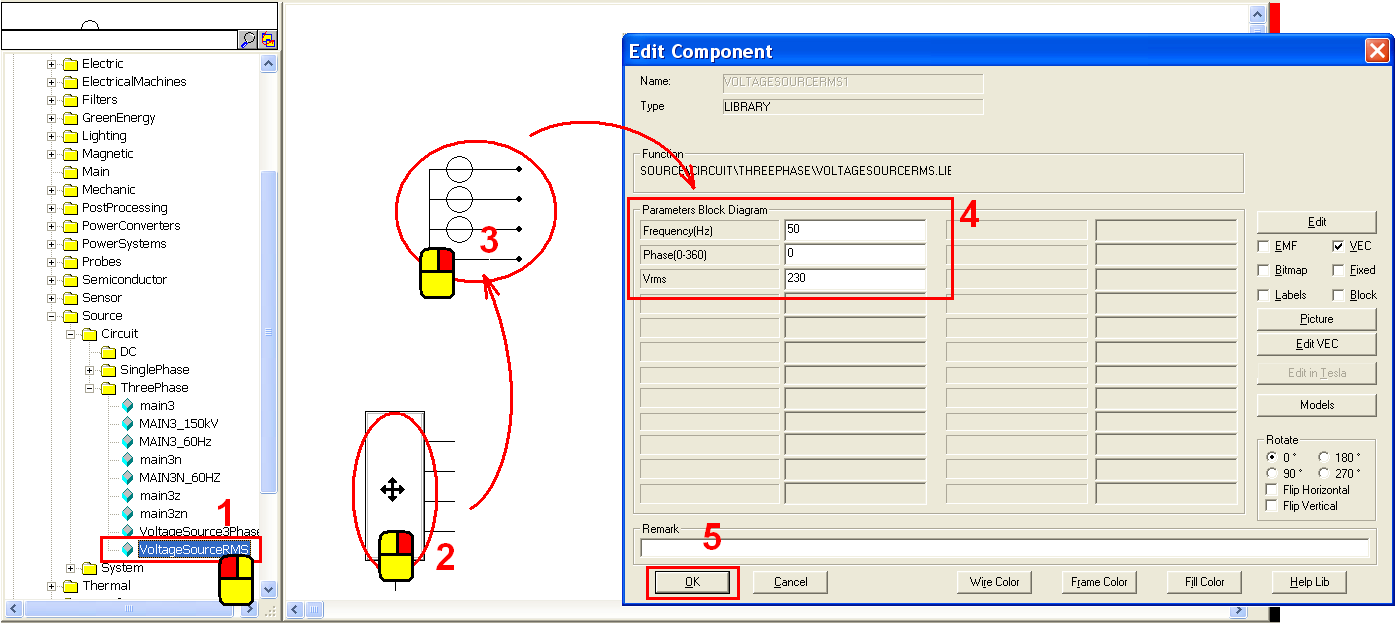To get the induction motor, left-click Components/Library/ElectricalMachine/IM/IM (step 1) and release the mouse. The induction motor follows the cursor and right-click to change its direction if needed (step 2). Left-click on the workscreen to put the induction motor in the right side of the AC voltage source. Right-click the induction motor to check its setting (step 3). In the pop-window, we can see the parameters of the induction motor (step 4). Click �Help Lib� to see the parameter explanation (step 5):

• Rs = 39m (stator resistance)
• Rr = 44m (stator resistance)
• f = 1u (friction of the rotor)
• J = 1m (rotor inertia)
• p = 2 (pole pairs)
• Ls = 0.6m (stator leakage inductance)
• Lr = 0.6m (rotor leakage inductance)
• Lm = 19.4m (stator magnetizing induction)

Click OK to save the setting (step 6).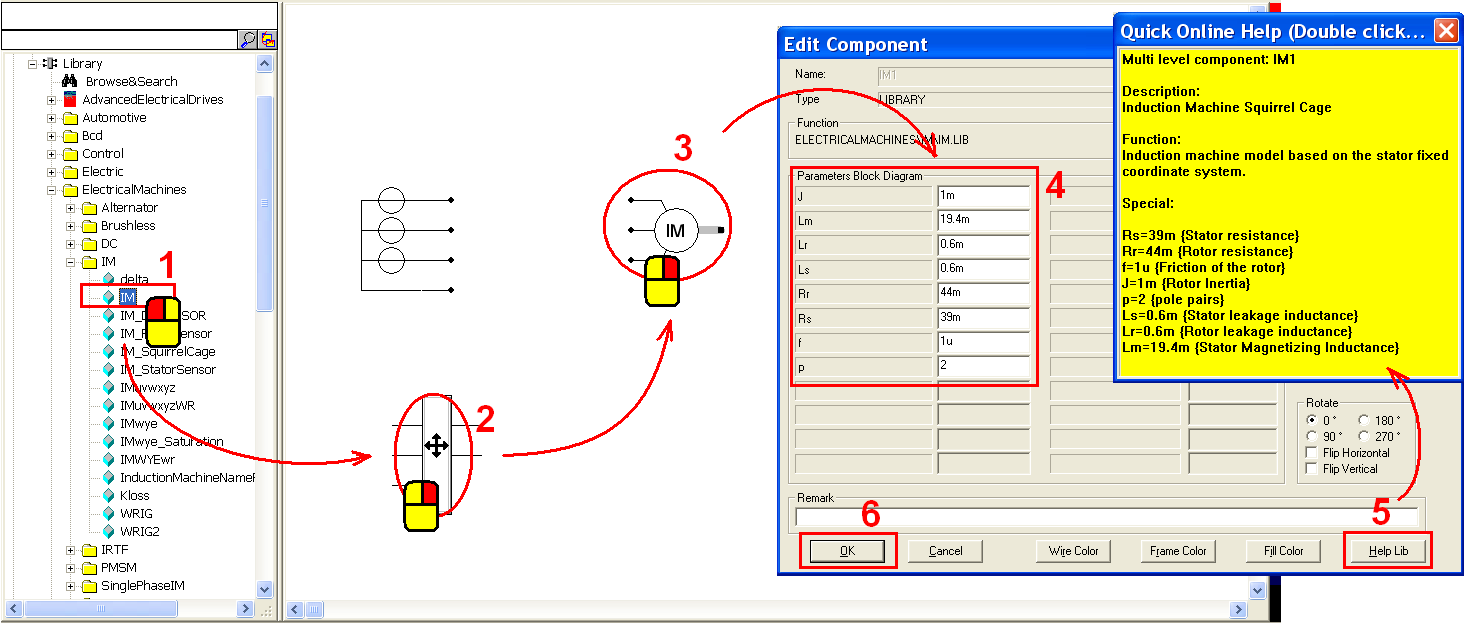Then get the variable mechanical load for the motor, left-click Components/Library/Mechanic/Rotational/LoadQuadratic (step 1). The quadratic load follows the cursor and right-click on it to change the direction if needed (step 2). While the angular velocity input of the quadratic load is leftward, left-click on the workscreen to put the quadratic load in the right side of the induction motor. Right-click the quadratic load (step 3) to change its parameters: J[Kgm�] = 1, Tn[Nm] = 60 and Wn[Rad/s] = 314.15927 (step 4). Click OK to save the setting (step 5).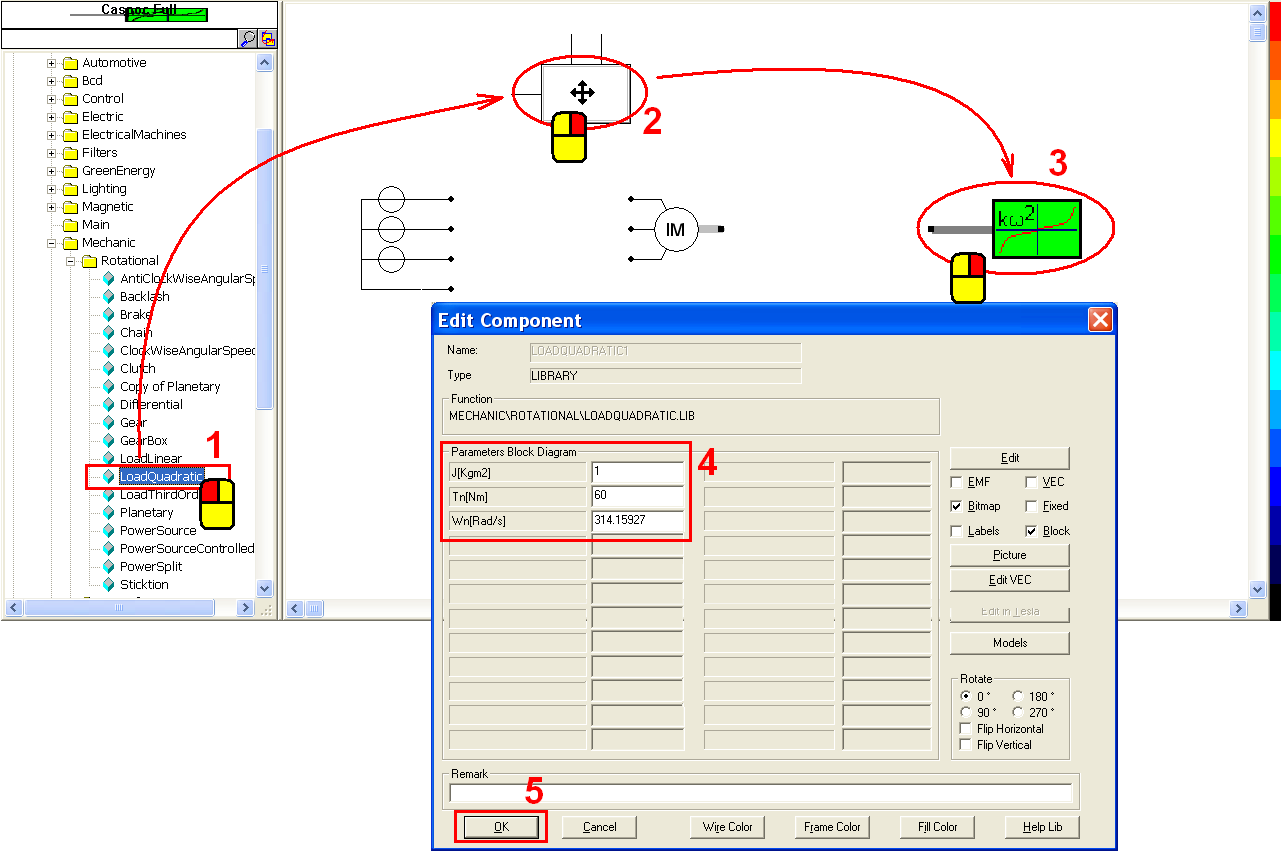Connect the AC voltage source, the induction motor and the quadratic load with the following configuration (step 1). Currently we have not set an electric ground. Right-click on the last round dot of the AC voltage source (step 2) to add a ground label. In the pop-window, enter Label = Ground (step 3) and click OK to save the setting (step 4).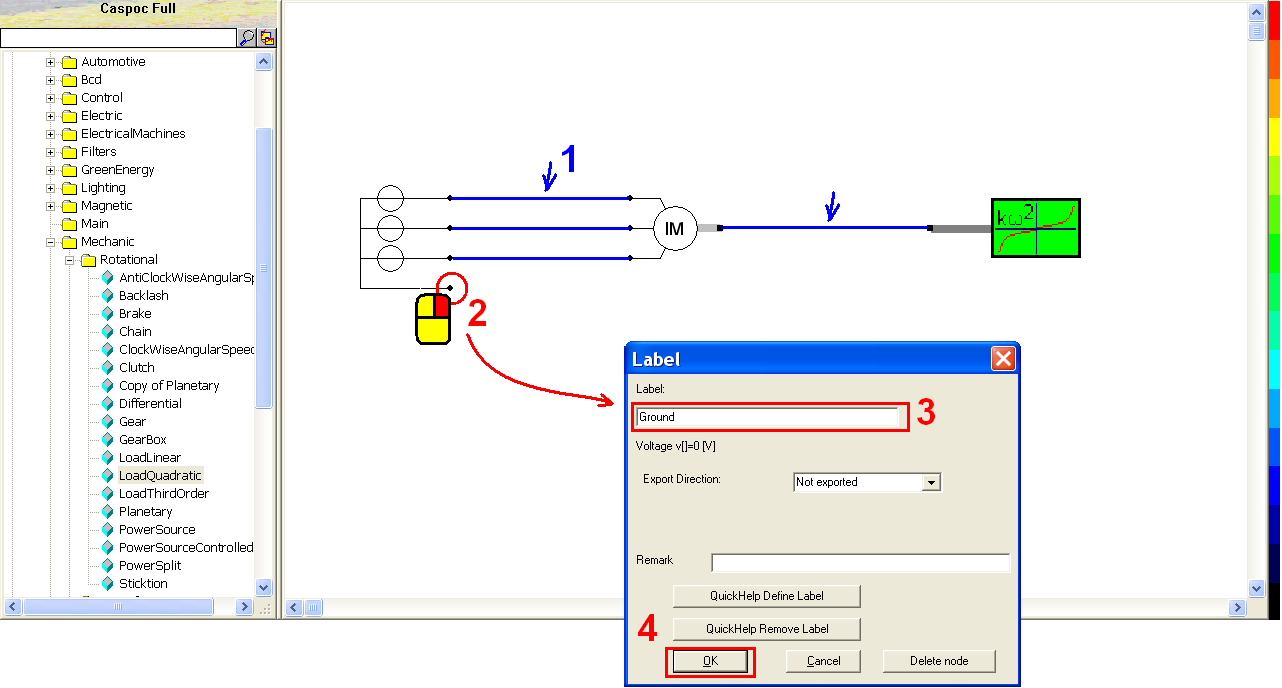Now we can start to run a simulation. Click the scope iconin the experience bar (step 1) to put a scope below the Induction motor to measure its angular velocity (step 2). Left-click the right-bottom corner of the scope and hold down the mouse to enlarge this scope (step 3). Then connect the angular velocity output of the induction motor to the first blue input trace (step 4).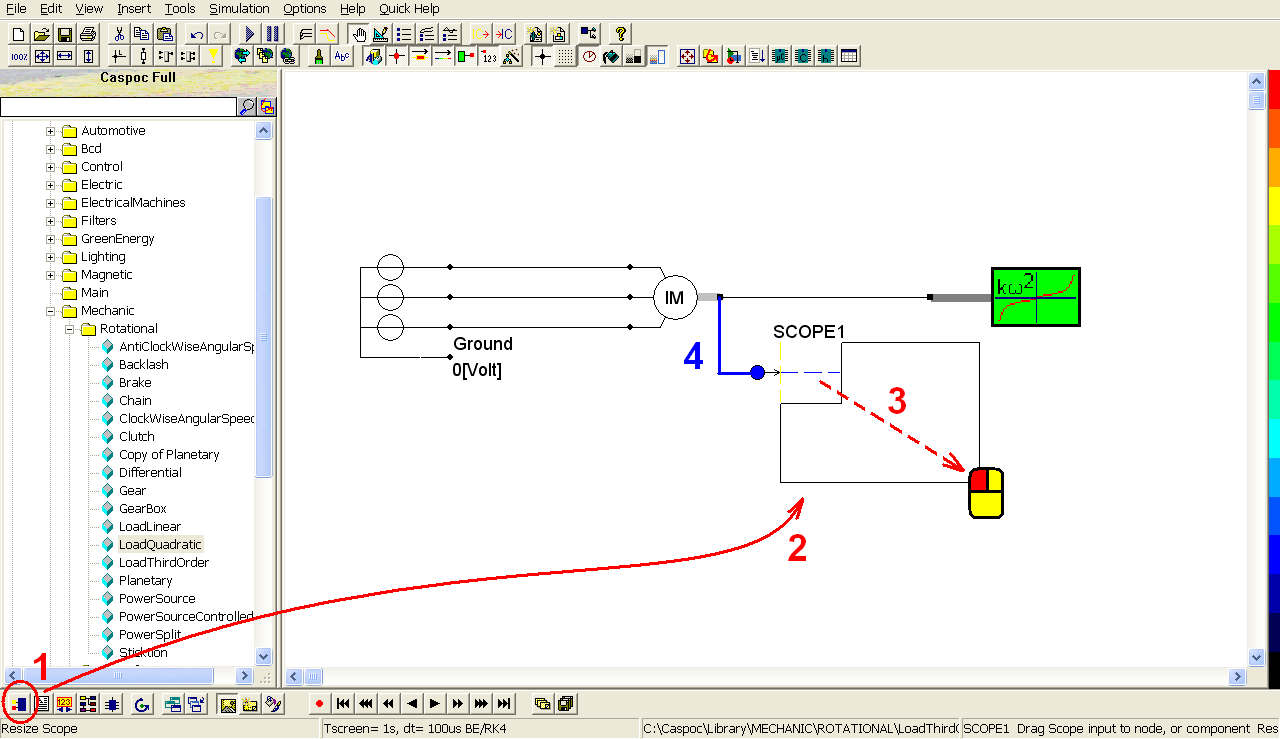Click the short-cut of simulation parameter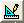(step 1). In the pop-window, select Euler for the Numerical Integration Method (step 2), Tscreen = 1, dt = 100u (step 3) and then click OK to save the setting (step 4).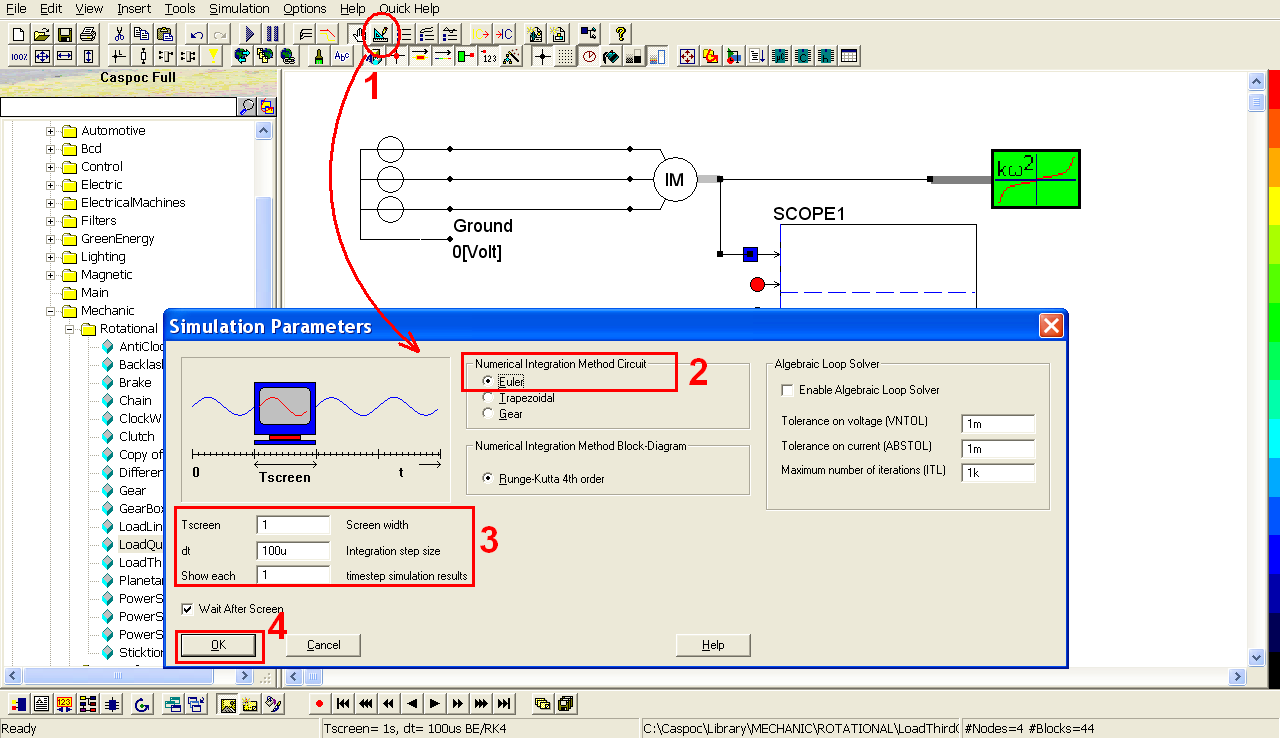Click the short-cut of start simulation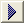(step 1). Right-click on the scope (step 2) to see the details of the simulation result (step 3). We can see the angular speed converges toward a certain speed after 500 milliseconds.But what if we want to observe the stators� currents? CASPOC provides plenty of sensors for measuring any information in the simulation. To put the current sensor, we have to delete the connection between the induction motor and the AC voltage source. Left-click and draw the cursor to select the three lines between them (step 1). Release the mouse and click Delete key on the keyboard to delete the three lines (step 2). Left-click Components/Library/Sensor/Current/i_3phase (step 3) and release the mouse. The current sensor follows the cursor and right-click to change its direction if needed. Then Left-click to put it on the workscreen (step 4). Make sure the current direction for the current sensor is rightward (step 5). If not, right-click the current sensor and modify its rotation parameter to 0 degree.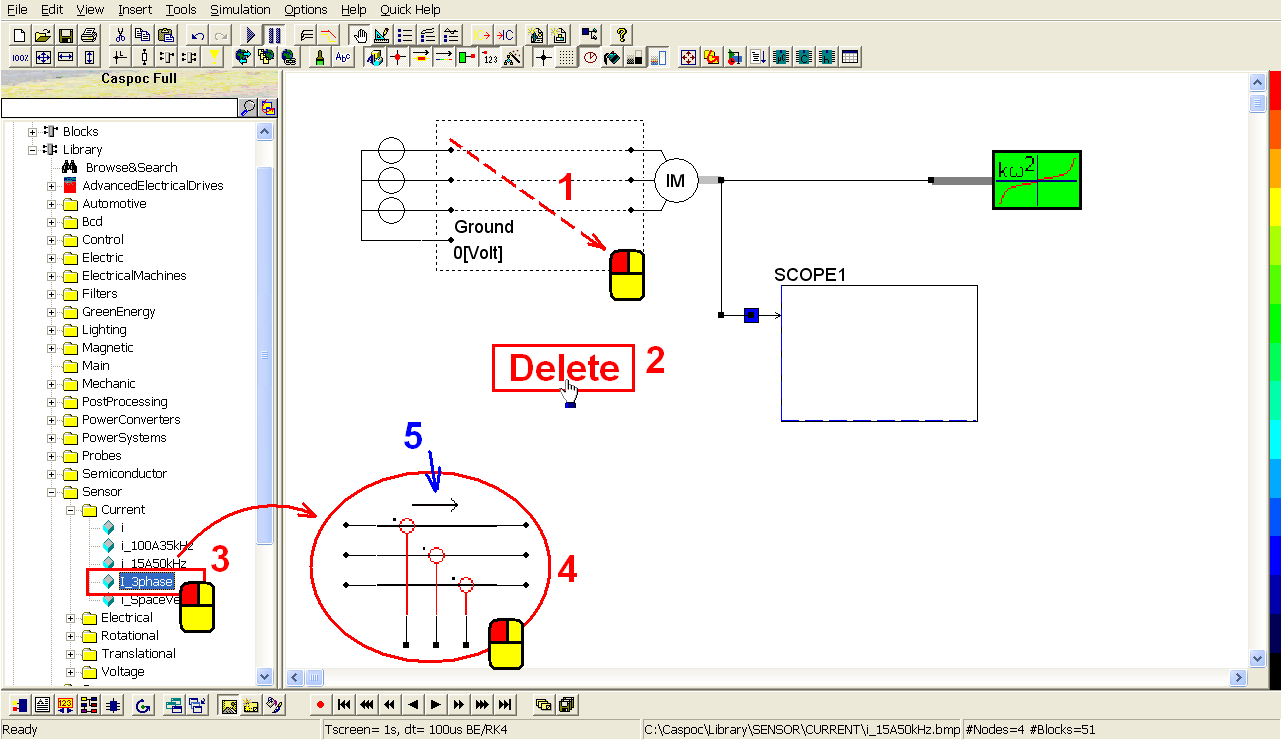Wherever the current sensor, left-click on it and hold down to put it between the voltage source and the induction motor (step 1). To get the measured current information from the current sensor, we need another scope. Click the scope iconin the experience bar (step 2) to put a scope below first scope. Left-click the right-bottom corner of the scope and hold down the mouse to enlarge this scope (step 3).Then connect the three square dots (the measured current outputs) to the three input traces of the second scope (step 1), shown in blue, red and green. Click the short-cut of start simulation(step 2).Right-click on the second scope (step 1) to see the details of the simulation result (step 2).Now add the inertia of the rotor to see how it affects the angular velocity. Left-click Components/Circuit/Rotational/INERTIA (step 1) and release the mouse. The inertia follows the cursor and right-click to change its direction if needed (step 2). Left-click on the line between the induction and the quadratic load to the inertia. Make sure the center of the inertia is exactly on the line (step 3).Click the short-cut of start simulation(step 1). Right-click on the first scope (step 2) to see the details of the simulation result (step 3). We can see the angular speed converges toward a certain speed after 800 milliseconds (longer than 500 milliseconds before adding the inertia). Right-click on the second scope (step 4) to see the details of the simulation result (step 5). The stator currents also need more time to converge to a certain value.Now we want to know how the rotor inertia affects the torque. To measure the torque, we need two torque sensors: one before the inertia, the other after. Left-click the quadratic load and hold down to move it to the right side (step 1), then release the mouse to fix its position. Left-click the inertia and hold down to move it to the right side (step 2), then release the mouse to fix its position. Hold down the Ctrl key and left-click the two part of the line to select them as below (step 3). Click Delete key to delete the selected line (step 4).Left-click Components/Library/Sensor/Rotational/PowerTorque (step 1) and release the mouse. Left-click to put the torque sensor with the induction motor (step 2). Similarly left-click again Components/Library/Sensor/Rotational/PowerTorque and release the mouse. Left-click to put the torque sensor with the quadratic load (step 3). Connect the two torque sensors with the rotor inertia (step 4).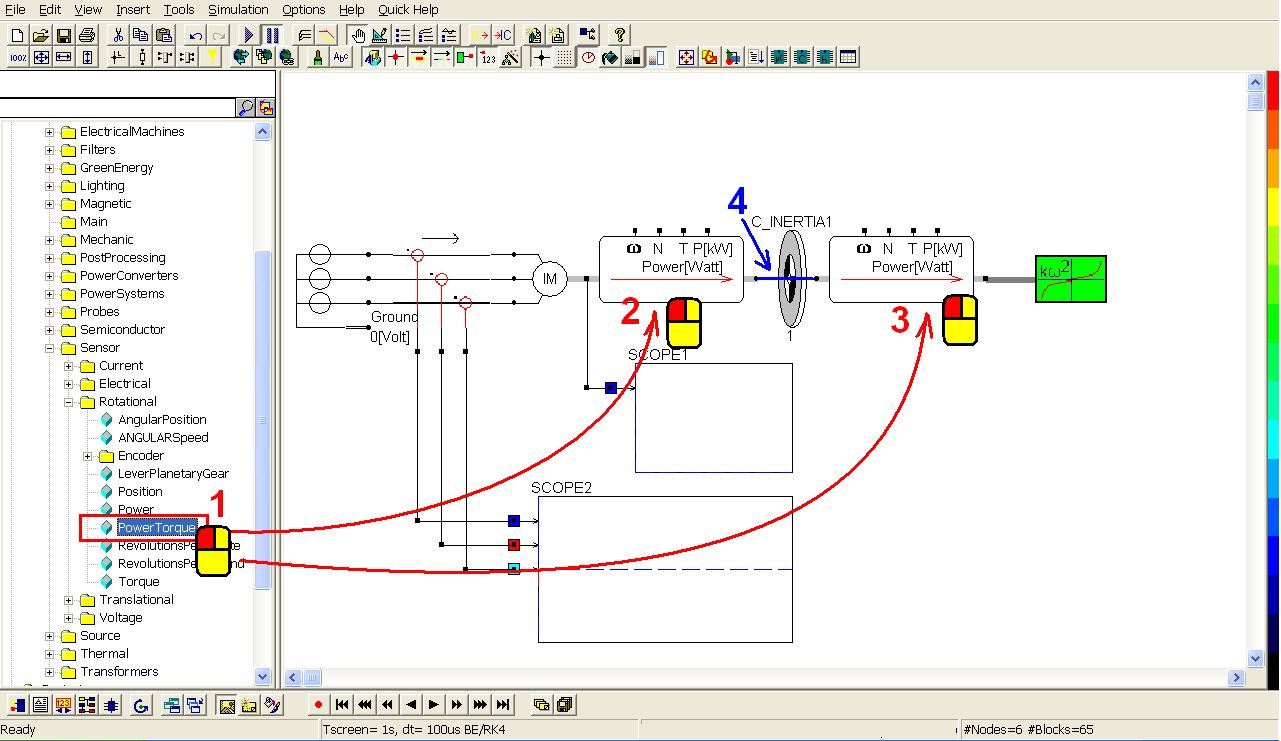To get the measured torque value, click the scope iconin the experience bar (step 1) to put the third scope above the quadratic load (step 2). Left-click the right-bottom corner of the scope and hold down the mouse to enlarge this scope (step 3). Then connect the rotational speed (N) and torque (T) outputs of the torque sensors to the four input traces (step 4).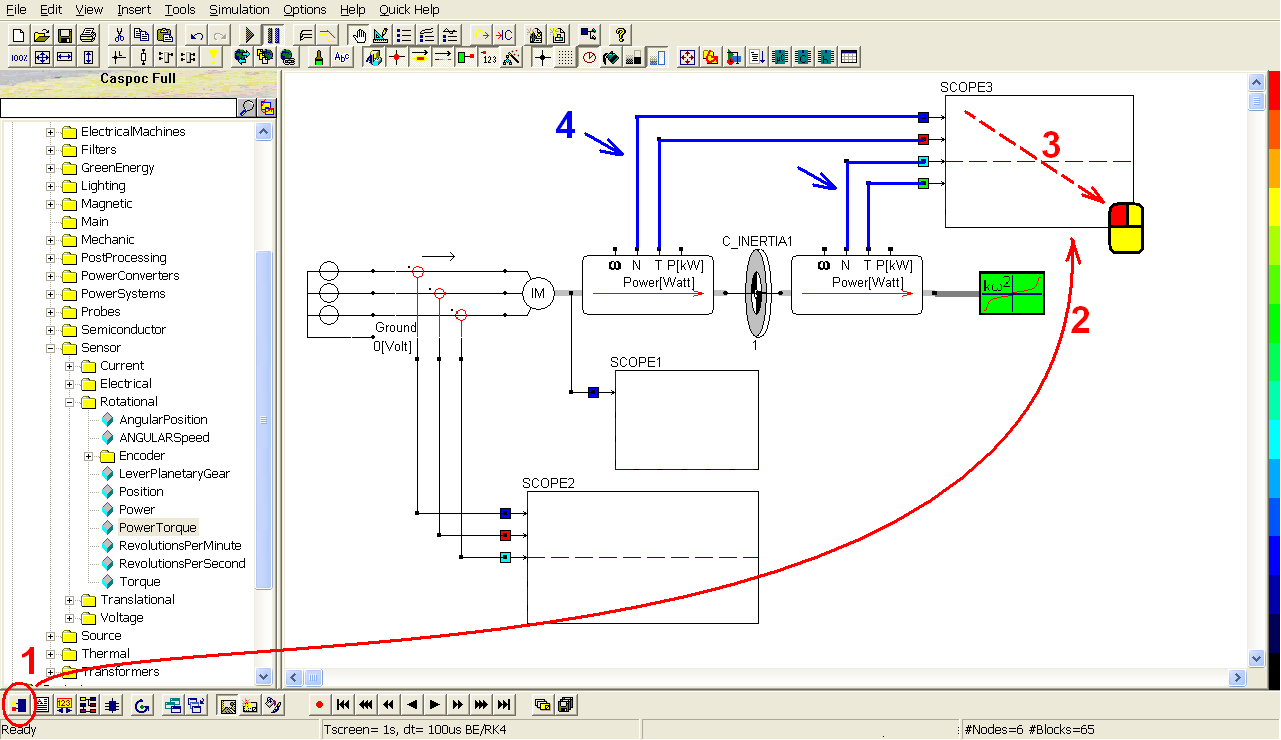Right-click on the third scope (step 1) to change the setting. Select View/XY Writer (step 2) and close the pop-window. As a result, the rotational speeds will be shown in x-axis, and torque in y-axis.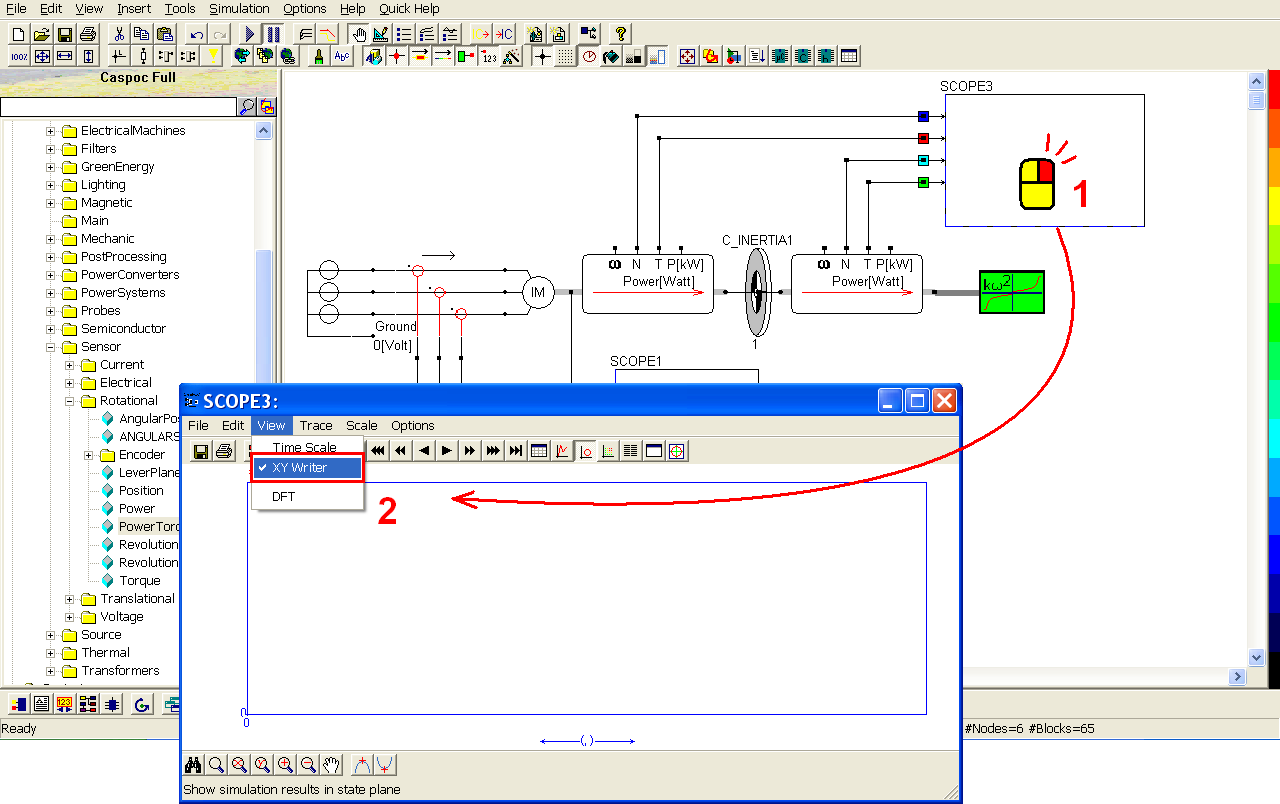Right-click the quadratic load to change some parameters (step 1). To see clearly the characteristic of the quadratic load and the affection of the rotor inertia, change the inertia of the load to a smaller value: J[kgm�] = 1u. Then to make the quadratic relation observably, increase the proportion of Tn and Wn: Tn[Nm] = 750 and Wn[Rad/s] = 156 (step 2). Click OK to save the setting (step 3).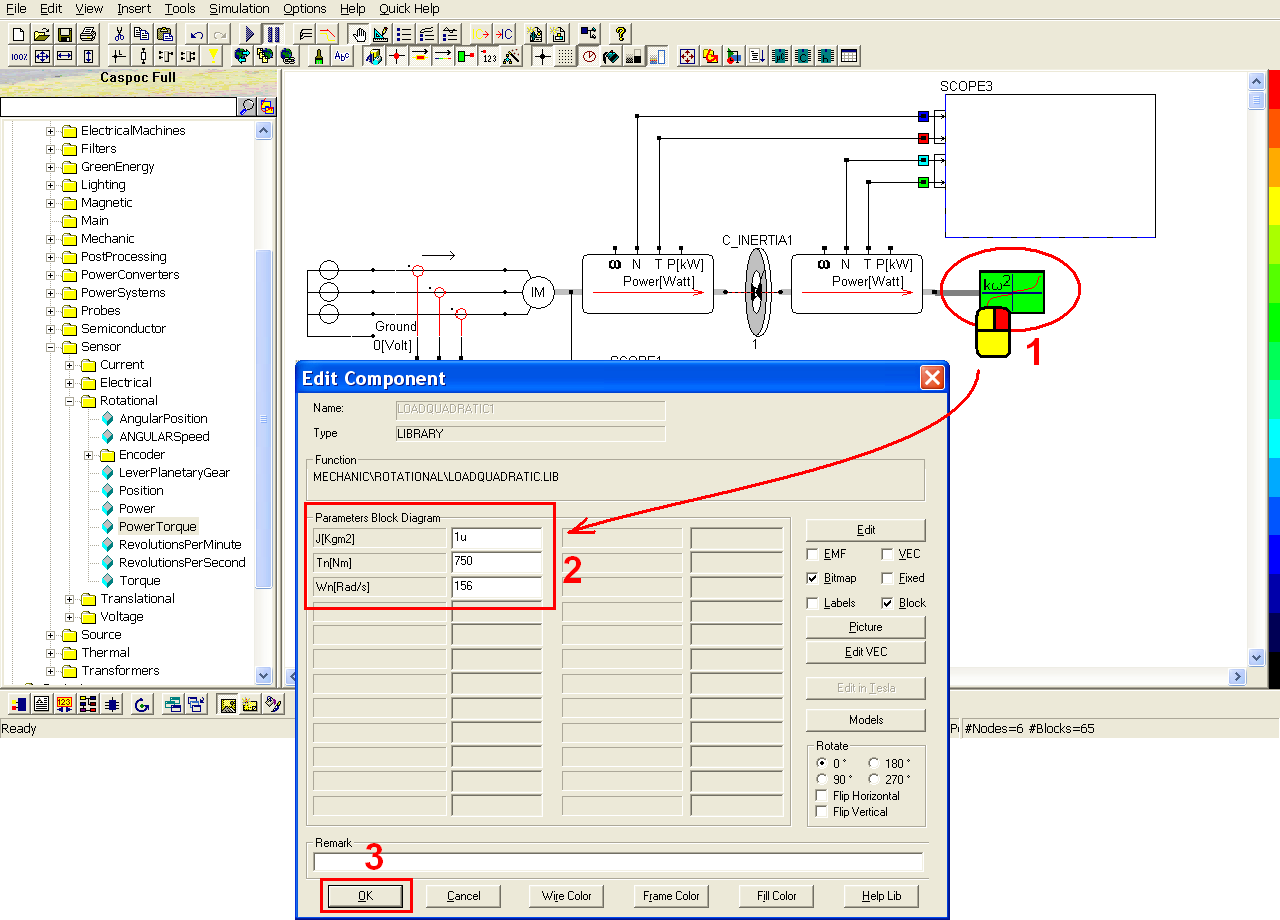Click the short-cut of start simulation(step 1). Right-click on the third scope (step 2) to see the details of the simulation result (step 3). We can see before the relation between the rotational speed (x-axis) and the torque (y-axis).